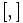# CS-Baer correspondence

## Definition

The CS-Baer correspondence (short for central series Baer correspondence) is a generalization of the Baer correspondence that operates as follows:

CS-Baer Lie group$G$ along with central subgroup$H$ in which every element of$[G,G]$ has a unique square root$\leftrightarrow$ CS-Baer Lie ring$L$ along with central subring$M$ in which every element of$[L,L]$ has a unique half

Here a CS-Baer Lie group$G$ is simply a group of nilpotency class two that admits a central subgroup$H$ as described above, and CS-Baer Lie ring$L$ is simply a Lie ring of nilpotency class two that admits a central subring$M$ as described above.

A special subcorrespondence is where$H = Z(G)$ and where$M = Z(L)$. These groups and Lie rings correspond with each other.

### From group to Lie ring

Suppose$G$ is a LCS-Baer Lie group and$H$ is a central subgroup of$G$ containing$[G,G]$ such that every element of$[G,G]$ has a unique square root in$H$. Then,$G$ has

Lie ring operation that we need to define Definition in terms of the group operations Further comments
Addition, i.e., define$x + y$ for$x,y \in G$$x + y := \frac{xy}{\sqrt{[x,y]}}$ Since$[x,y] \in [G,G]$, it has a unique square root in$H$. We denote this unique square root as$\sqrt{[x,y]}$. Note that$H$ is in the center, hence the element$\sqrt{[x,y]}$ is central. Thus, to divide by it, we do not need to specify whether we are dividing on the left or on the right.
Identity element for addition, denoted$0$. Same as identity element for group multiplication, denoted$e$ or$1$. This automatically follows from the way addition is defined.
Additive inverse, i.e., define$-x$ for$x \in G$. Same as$x^{-1}$, i.e., the multiplicative inverse in the group. This automatically follows from the way addition is defined.
Lie bracket, i.e., the$[ , ]$ map in the Lie ring. Same as the group commutator$[x,y] = xyx^{-1}y^{-1}$.